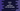# Kotlin example to use aggregate with groupingBy## Introduction :

groupingBy method is used to group items of a collection using a keySelector function. It returns one Grouping object. For example, we can group a list of strings by the first character of each string. aggregate is another function that can be used with a Grouping to apply one operation on each element of each group sequentially. In this post, I will show you how to use groupingBy with one example.

### Syntax of aggregate :

The syntax of aggregate method is as below :

``````public inline fun <T, K, R> Grouping<T, K>.aggregate(
operation: (key: K, accumulator: R?, element: T, first: Boolean) -> R
): Map<K, R> {
return aggregateTo(mutableMapOf<K, R>(), operation)
}``````

It applies the operation on each element of each group sequentially. It passes the previously accumulated value and the current value. Following are the meanings of each parameter :

• key : This is the key of the group
• accumulator : This is the accumulator. It is null for the first element. We need to create the accumulator for the first element.
• element : Current element.
• first : Boolean value to define if it is the first element or not. It is useful to create the accumulator.

### Example program :

Let me show you one example of how it works. In this example, we have one list of strings. We will group the strings by the first character of each string and separate each value of each group by a comma.

``````fun main() {
val intGroup = listOf("Clara", "Alex", "Bob", "Ava", "Bennett", "Camila")

val data = intGroup.groupingBy { it.first() }.aggregate { key, accumulator: StringBuilder?, element, first ->
if (first)
StringBuilder().append("Names Starts with \$key: ").append(element)
else
accumulator!!.append(",").append(element)
}

print(data.values)

}``````

Here, the accumulator is a StringBuilder. The StringBuilder is created for the first element. We are appending the key and elements to the StringBuilder. It will print the below output :

``[Names Starts with C: Clara,Camila, Names Starts with A: Alex,Ava, Names Starts with B: Bob,Bennett]``